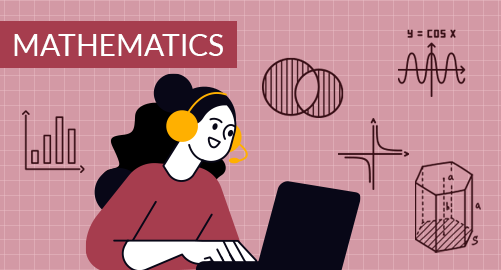#### through a demo today# Year 4 Mathematics

Mathematics
Year 4

The Year 4 Mathematics curriculum offers students a strong foundation in essential mathematical skills and concepts, setting the stage for more advanced math adventures ahead. This grade covers a range of important topics, including numbers, factors and multiples, fractions, decimals, financial mathematics, geometry, measurements, data handling, time and location. As students progress through this curriculum, they will gain a deeper grasp of mathematical principles and problem-solving strategies, empowering them to use math effectively in everyday situations.

## What you'll learn

• 1

Number System

• 2

Factors and Multiples

• 3

Fractions and Decimals

• 4

Financial Mathematics

• 5

Geometry

• 6

Measurements

• 7

Data Handling and Probability

• 8

Time and Location

## Skills you'll learnNumeracy Skills: Distinguish between different types of numbers, develop a strong grasp of place value, proficiency in fundamental arithmetic operations, acquire the ability to compare numbers, breaking complex problems in a simple wayProblem-Solving Skills: Understanding number relationships, calculating LCM and HCF, applying divisibility rules, solving various real-life problems that involve doublingProblem-Solving Skills: Handle real-world problems involving money, measurements, percentages, categorising fractions, ranking numerical values, and practical scenarios like cooking measurements and financial calculationsFinancial Literacy: Accurately count various denominations of currency, handle international transactions and understand currency conversion rates, rounding off money helps to estimate and simplify financial calculationsGeometrical Skills and Spatial Relationships: Proficiency in measuring angles, properties of 2-D and 3-D shapes, creating geometric patterns, recognising symmetrical figures, visualising the relationships between 2-D and 3-D shapesPractical Skills: Calculate the fence required, measure boundaries, find the material required through the area, measure liquid quantities and temperaturesData Analysis and Predictions: Data organisation, create graphs, interpret data, calculate predictionsCritical Thinking Skills: Plan and schedule activities, estimate the time, understand the passage of time, locate the positions, estimate travel times